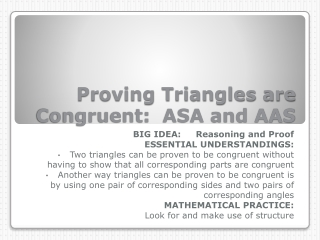# Proving Triangles are Congruent: ASA and AAS - PowerPoint PPT PresentationDownload PresentationProving Triangles are Congruent: ASA and AAS

Proving Triangles are Congruent: ASA and AASDownload Presentation## Proving Triangles are Congruent: ASA and AAS

- - - - - - - - - - - - - - - - - - - - - - - - - - - E N D - - - - - - - - - - - - - - - - - - - - - - - - - - -
##### Presentation Transcript

1. Proving Triangles are Congruent: ASA and AAS BIG IDEA: Reasoning and Proof ESSENTIAL UNDERSTANDINGS: Two triangles can be proven to be congruent without having to show that all corresponding parts are congruent Another way triangles can be proven to be congruent is by using one pair of corresponding sides and two pairs of corresponding angles MATHEMATICAL PRACTICE: Look for and make use of structure

2. The postulate and theorem in this lesson allow students to prove that two triangles are congruent based on two pairs of congruent angles and one pair of congruent sides. • These postulates and theorem represent the minimum number of conditions necessary to prove triangle congruence. • As in other lessons, the names of congruence postulates and theorems are reminders about the position of the congruent corresponding parts. Math Background

3. ANGLE-SIDE-ANGLE (ASA) CONGRUENCE POSTULATE: • If _______________ angles and the _______________ side of one triangle are _______________ to two _______________ and the included _______________ of a second triangle, then the two triangles are _______________ • ANGLE-ANGLE-SIDE (AAS) CONGRUENCE THEOREM: • If _______________ angles and the _______________ side of one triangle are _______________ to two _______________ and the corresponding nonincluded _______________ of a second triangle, then the two triangles are ____________ CONGRUENCE POSTULATES

4. 1. • 2. EX: Decide whether enough information is given to prove that the triangles are congruent. If there is enough information, state the congruence postulate you would use.

5. 3. • 4. EX: Decide whether enough information is given to prove that the triangles are congruent. If there is enough information, state the congruence postulate you would use.

6. 5. • 6. EX: Decide whether enough information is given to prove that the triangles are congruent. If there is enough information, state the congruence postulate you would use.

7. EX 7: GIVEN:PROVE:

8. EX 8: GIVEN:PROVE:

9. EX 9: GRAPH THE EQUATIONS IN THE SAME COORDINATE PLANE. LABEL THE VERTICES OF THE TWO TRIANGLES FORMED BY THE LINES. SHOW THAT THE TRIANGLES ARE CONGRUENT